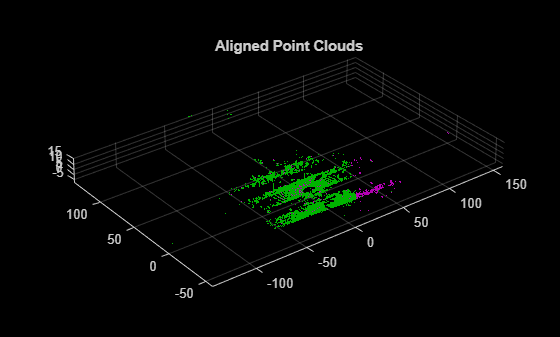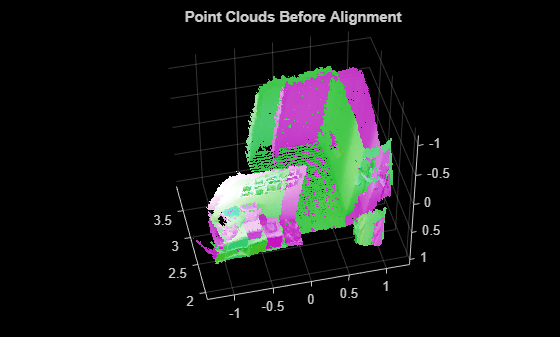# detectISSFeatures

Detect ISS feature points in point cloud

Since R2022a

## Syntax

``points = detectISSFeatures(ptCloud)``
``[points,indices] = detectISSFeatures(ptCloud)``
``___ = detectISSFeatures(ptCloud,Name=Value)``

## Description

example

````points = detectISSFeatures(ptCloud)` detects intrinsic shape signature (ISS) feature points in the input point cloud `ptCloud`. ISS is a 3-D shape representation method for 3-D object recognition. ISS feature points are the points rich in 3-D structural variation in their neighbourhoods. ```
````[points,indices] = detectISSFeatures(ptCloud)` additionally returns the linear indices for the detected ISS feature points.```
````___ = detectISSFeatures(ptCloud,Name=Value)` specifies options using one or more name-value arguments in addition to any combination of output arguments from previous syntaxes. For example, `detectISSFeatures(ptCloud,Radius=0.05)` computes the ISS saliency within a 0.05 m radius around each point when identifying the feature points.```

## Examples

collapse all

Read a point cloud from a PLY file into the workspace.

`ptCloud = pcread("teapot.ply");`

Detect ISS feature points in the point cloud, and display the point cloud and ISS feature points.

```points = detectISSFeatures(ptCloud); figure(Name="Detected feature points") pcshow(ptCloud) hold on plot3(points(:,1),points(:,2),points(:,3),"pentagram", ... MarkerSize=5,MarkerFaceColor=[1 0.6 0.6],Color="red")```Read a point cloud from a PCD file into the workspace.

`ptCloud = pcread("highwayScene.pcd");`

Transform the input point cloud by applying both rotation and translation.

```eulerAngles = [0 0 30]; trans = [5 5 0]; tform = rigidtform3d(eulerAngles,trans); ptCloudTform = pctransform(ptCloud,tform);```

Detect ISS feature points in the original, fixed, and transformed, moving point clouds.

```[~,indicesFixed] = detectISSFeatures(ptCloud); [~,indicesMoving] = detectISSFeatures(ptCloudTform);```

Extract FPFH features for the detected feature points in each point cloud.

```ptCloudFixed = select(ptCloud,indicesFixed); fixedFeature = extractFPFHFeatures(ptCloudFixed); ptCloudMoving = select(ptCloudTform,indicesMoving); movingFeature = extractFPFHFeatures(ptCloudMoving);```

Match the features between the fixed and moving point clouds.

```[matchingPairs,scores] = pcmatchfeatures(fixedFeature,movingFeature, ... ptCloudFixed,ptCloudMoving,Method="Exhaustive");```

Estimate the transform using the matched features.

```fixedPts = select(ptCloudFixed,matchingPairs(:,1)); matchingPts = select(ptCloudMoving,matchingPairs(:,2)); estimatedTform = estgeotform3d(fixedPts.Location, ... matchingPts.Location,"rigid");```

Align the point clouds using the estimated transform.

`ptCloudAligned = pctransform(ptCloudTform,invert(estimatedTform));`

Visualize the aligned point clouds.

```pcshowpair(ptCloud,ptCloudAligned) title("Aligned Point Clouds")```Load a MAT file that contains point cloud data into the workspace. Extract the first two point clouds from the data.

```inputData = load("livingRoom.mat"); movingPtCloud = inputData.livingRoomData{1}; fixedPtCloud = inputData.livingRoomData{2};```

Visualize the extracted point clouds.

```pcshowpair(movingPtCloud,fixedPtCloud,VerticalAxis="Y",VerticalAxisDir="Down") title("Point Clouds Before Alignment")```Detect ISS feature points in the point clouds.

```[~,indicesMoving] = detectISSFeatures(movingPtCloud); [~,indicesFixed] = detectISSFeatures(fixedPtCloud);```

Extract the feature points from each point cloud, and register the point clouds to one another.

```movingISSPtCloud = select(movingPtCloud,indicesMoving); fixedISSPtCloud = select(fixedPtCloud,indicesFixed); tform = pcregistericp(movingISSPtCloud,fixedISSPtCloud, ... Extrapolate=true);```

Align the point clouds using the registered transform, and visualize the results.

```movingPtCloudAligned = pctransform(movingPtCloud,tform); figure pcshowpair(movingPtCloudAligned,fixedPtCloud,VerticalAxis="Y",VerticalAxisDir="Down") title("Aligned Point Clouds")```## Input Arguments

collapse all

Input point cloud, specified as a `pointCloud` object.

### Name-Value Arguments

Specify optional pairs of arguments as `Name1=Value1,...,NameN=ValueN`, where `Name` is the argument name and `Value` is the corresponding value. Name-value arguments must appear after other arguments, but the order of the pairs does not matter.

Example: `detectISSFeatures(ptCloud,Radius=0.05)` computes the ISS saliency within a 0.05 m radius around each point when identifying the feature points.

Neighborhood radius for computing ISS saliency, specified as positive scalar. The ISS saliency of a point is a measure of the richness of 3-D structural variation in its neighborhood, which determines whether or not the point is a feature point. The function computes a scatter matrix within the specified radius of each point to determine its ISS saliency and identify feature points. The default value is six times the average distance from each point in the input point cloud to its nearest neighbouring point. Units are in meters.

Data Types: `single` | `double` | `int8` | `int16` | `int32` | `int64` | `uint8` | `uint16` | `uint32` | `uint64`

Neighborhood radius in which to apply the non-maxima suppression algorithm, specified as positive scalar. The default value is four times the average distance from each point in the input point cloud to its nearest neighbouring point. Increasing this value can reduce the number of detected feature points. Units are in meters.

Note

`NonMaxRadius` value must be less than or equal to the value of `Radius`. Otherwise, the function reduces the value of `NonMaxRadius` to the value of `Radius`.

Data Types: `single` | `double` | `int8` | `int16` | `int32` | `int64` | `uint8` | `uint16` | `uint32` | `uint64`

Ratio of the second eigenvalue to first eigenvalue in the scatter matrix, specified as a positive scalar in the range (`0, 1`]. The function uses this ratio to define the x-, y-, z-axes of the intrinsic reference frame. For a lower ratio value, the function excludes the points with similar 3-D features along the first and second principal axes.

Data Types: `single` | `double` | `int8` | `int16` | `int32` | `int64` | `uint8` | `uint16` | `uint32` | `uint64`

Ratio of the third eigenvalue to second eigenvalue in the scatter matrix, specified as a positive scalar in the range (`0, 1`]. The function uses this ratio to define the x-, y-, z-axes of the intrinsic reference frame. For a lower ratio value, the function excludes the points with similar 3-D features along the second and third principal axes.

Data Types: `single` | `double` | `int8` | `int16` | `int32` | `int64` | `uint8` | `uint16` | `uint32` | `uint64`

Minimum number of neighbors required for an ISS feature point, specified as a positive integer. Increasing this value can reduce the total number of feature points detected.

Data Types: `single` | `double` | `int8` | `int16` | `int32` | `int64` | `uint8` | `uint16` | `uint32` | `uint64`

## Output Arguments

collapse all

ISS feature points in the input point cloud, returned as an M-by-3 matrix. M is the total number of feature points. Each row contains the [x y z] coordinates of a feature point.

Linear indices for the detected ISS feature points, returned as an M-by-1 matrix.

## Algorithms

Intrinsic shape signatures (ISS) are a method of 3-D shape representation. ISS feature points are rich in 3-D structural variations in their neighbourhood. This method has applications in modeling, visualization, and classification of 3-D point clouds.

To detect ISS feature points in a point cloud, the `detectISSFeatures` function follows these steps.

• Computes a point scatter matrix within the specified `Radius` around each point.

• Computes the eigenvalues λ1, λ2, and λ3 in decreasing order of magnitude for the scatter matrix. These eigenvalues represent a direction in the 3-D space based on the number of point position variations.

• Using the eigenvalues, the function defines a view-independent intrinsic reference frame with the principal x-, y-, z-axes.

• Uses λ2/λ1, λ3/λ2 as criteria to avoid the points with similar spatial spread along the principal axes while detecting feature points. You can specify eigenvalue ratios for λ2/λ1 and λ3/λ2 using the `MaxGamma21` and `MaxGamma32` arguments, respectively.

• Computes the ISS saliency for each point using the smallest eigenvalue, λ3. ISS feature point is the point with maximum ISS saliency within the specified radius around each point.

• You can further process these feature points to match point clouds, estimate pose transformations, and detect 3-D objects.

## Version History

Introduced in R2022a